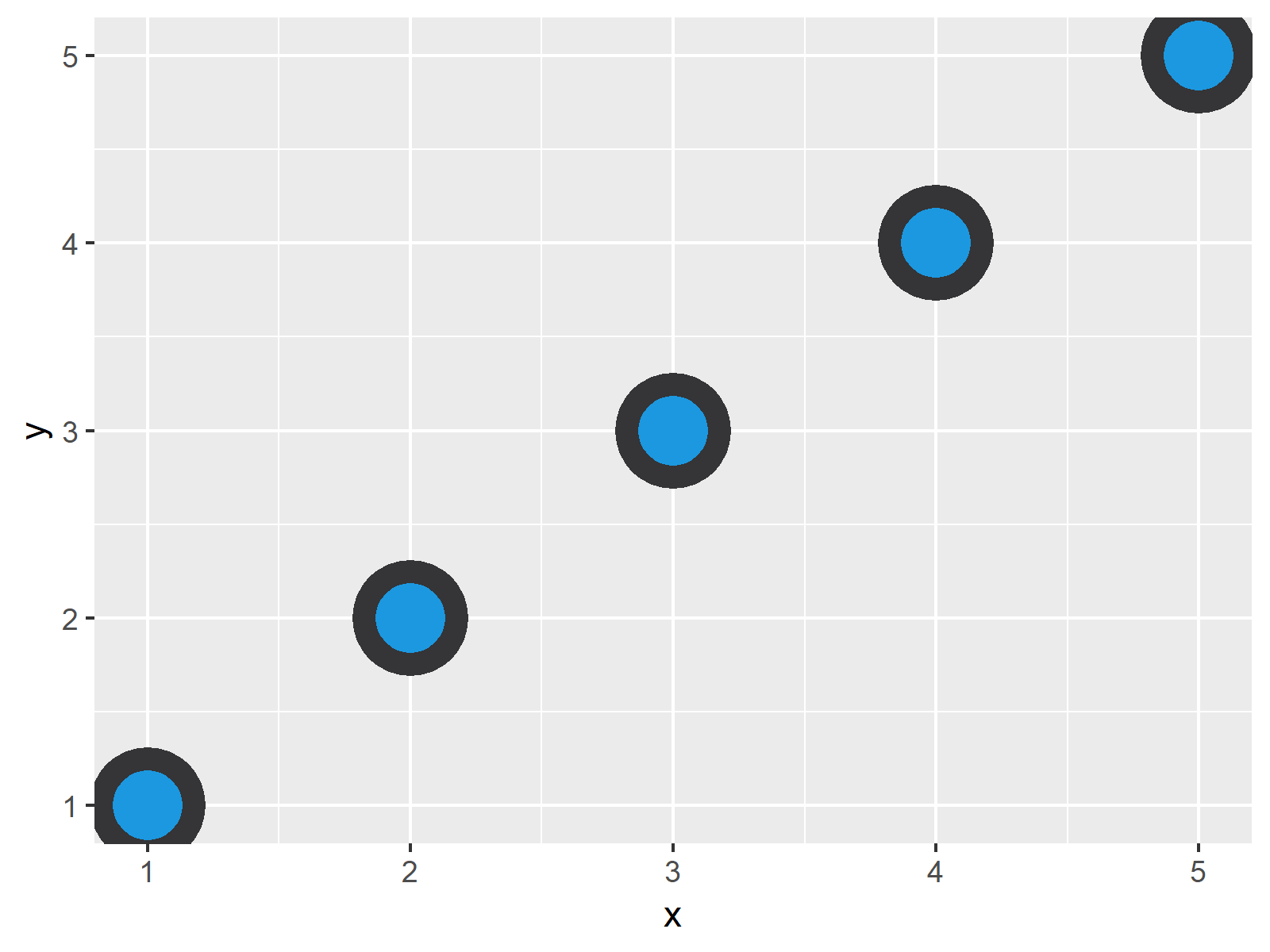# Control Point Border Thickness of ggplot2 Scatterplot in R (Example)

This article explains how to modify the thickness of point borders in a ggplot2 scatterplot in R programming.

The post will consist of this content:

Let’s just jump right in…

## Example Data, Packages & Basic Graphic

First, we’ll need to create some example data:

```data <- data.frame(x = 1:5,        # Create example data
y = 1:5)
data                               # Print example data
#   x y
# 1 1 1
# 2 2 2
# 3 3 3
# 4 4 4
# 5 5 5```

The previous output of the RStudio console shows the structure of our example data: It consists of five rows and two numeric columns.

We also have to install and load the ggplot2 package, if we want to use the corresponding functions:

```install.packages("ggplot2")        # Install ggplot2 package

Now, we can plot our data as follows:

```ggplot(data, aes(x, y)) +          # Create scatterplot
geom_point(fill = "#1b98e0",
color = "#353436",
size = 10,
shape = 21)```Figure 1 illustrates the output of the previous syntax – A ggplot2 Scatterplot with relatively thin borders.

## Example: Increasing Thickness of ggplot2 Point Borders Using stroke Argument

In this Example, I’ll explain how to control the border size of a ggplot2 xyplot. Have a look at the following R code – We are simply adding the stroke argument within the geom_point function. The larger the stroke argument gets, the thicker are the point borders. Let’s run the code:

```ggplot(data, aes(x, y)) +          # Increase thickness of point borders
geom_point(fill = "#1b98e0",
color = "#353436",
size = 10,
shape = 21,
stroke = 5)```As shown in Figure 2, we created a ggplot2 graph showing points with thick borders.

## Video & Further Resources

Would you like to learn more about the modification of points in ggplot2 plots? Then you may watch the following video of my YouTube channel. In the video, I’m explaining the R programming codes of this article in a live session:

Please accept YouTube cookies to play this video. By accepting you will be accessing content from YouTube, a service provided by an external third party.If you accept this notice, your choice will be saved and the page will refresh.

Furthermore, you might want to read some of the related articles of this website.

At this point you should know how to change the border thickness of points in a ggplot2 graphic in the R programming language. In case you have further questions, let me know in the comments section.

Subscribe to the Statistics Globe Newsletter